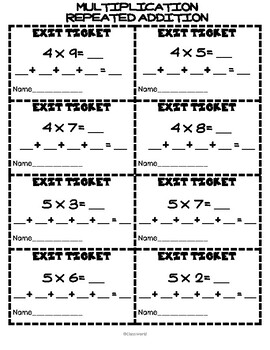# EXIT TICKETS MATH/Multiplication properties/ Multiplication strategiesSubject
Resource Type
Format
PDF (1 MB|15 pages)
Standards
\$1.50
\$1.50

### Description

This material is great to finish your multiplication classes. In which you teach multiplication strategies or their properties.

Give a ticket to each student, give them a few minutes to cover and deliver. This way you can have feedback on how your students are doing.

COMMON CORE STANDARDS

CCSS.MATH.CONTENT.2.OA.C.3

CCSS.MATH.CONTENT.3.OA.A.1

CCSS.MATH.CONTENT.3.OA.B.5

This pack includes 53 tickets:

• Multiplication grouping.
• Multiplication with array.
• Multiplication with sum.
• Commutative property.
• Associative property.
• Distributive property.
Total Pages
15 pages
N/A
Teaching Duration
30 minutes
Report this Resource to TpT
Reported resources will be reviewed by our team. Report this resource to let us know if this resource violates TpT’s content guidelines.

### Standards

to see state-specific standards (only available in the US).
Apply properties of operations as strategies to multiply and divide. Examples: If 6 × 4 = 24 is known, then 4 × 6 = 24 is also known. (Commutative property of multiplication.) 3 × 5 × 2 can be found by 3 × 5 = 15, then 15 × 2 = 30, or by 5 × 2 = 10, then 3 × 10 = 30. (Associative property of multiplication.) Knowing that 8 × 5 = 40 and 8 × 2 = 16, one can find 8 × 7 as 8 × (5 + 2) = (8 × 5) + (8 × 2) = 40 + 16 = 56. (Distributive property.)
Interpret products of whole numbers, e.g., interpret 5 × 7 as the total number of objects in 5 groups of 7 objects each. For example, describe a context in which a total number of objects can be expressed as 5 × 7.
Use addition to find the total number of objects arranged in rectangular arrays with up to 5 rows and up to 5 columns; write an equation to express the total as a sum of equal addends.
Determine whether a group of objects (up to 20) has an odd or even number of members, e.g., by pairing objects or counting them by 2s; write an equation to express an even number as a sum of two equal addends.